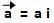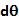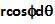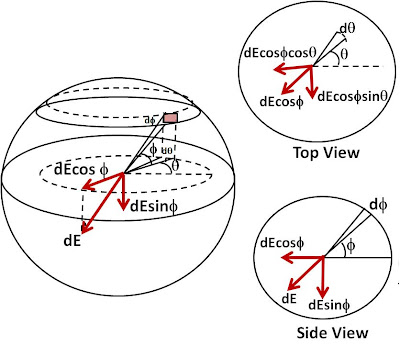## Saturday, February 6, 2010

### Irodov Problem 3.16The easiest way to solve this problem is to choose a coordinate system where the x-axis is oriented in the direction of the vector. In such a coordinate system the vectorcan be simply written as. The charge density of the sphere is then given by,Now any point on the surface of the sphere can be represented by two angles, namely the azimuthal angleand the inclination angleas shown in the figure. The coordinates of this point are then given by,Now consider an infinitesimally small rectangular section on the sphere that subtends azimuthal and inclination angles ofandrespectively. The dimensions of the rectangle are given byandas shown in the figure. The area of this rectangle is thus given by,The charge contained in this infinitesimally small section of the sphere surface is given by,The electric field due to this infinitesimally small section at the center is given by,Now recall that we had chosen a coordinate system where the x-axis was oriented along the vector. From the above solution it is clear that E is oriented along the x-axis, i.e. in the direction of. Hence, in general for a coordinate system the electric field can be written as,